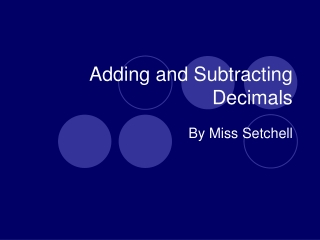DownloadDownload PresentationTélécharger la présentation- - - - - - - - - - - - - - - - - - - - - - - - - - - E N D - - - - - - - - - - - - - - - - - - - - - - - - - - -
##### Presentation Transcript

1. Adding and Subtracting Decimals By Miss Setchell

2. Tenths Hundredths Thousandths Review of DecimalPlace Value 0 .2 3 4

3. Hundredths 0 .7 9 8 Practice

4. Thousandths 0 .1 0 6 Practice

5. Tenths 0 .1 0 6 Practice

6. 3,984+2,827 Review of Adding Whole Numbers 1 1 1 1 1 27+86 182+376 1 1 3 6 , 8 1 1 5 5 8

7. 9,237- 5,338 Review of Subtracting Whole Numbers 1 1 1 8 1 3 4 1 1 2 1 8 95- 26 456- 188 6 9 3 , 8 9 9 2 6 8

8. 3.984+2.827 Adding Decimals 1 1 1 1 1 2.7+8.6 1.82+3.76 . 1 1 3 . . 5 5 8 6 8 1 1

9. 9.237- 5.338 Subtracting Decimals 1 8 1 3 4 1 1 1 1 2 1 8 9.5- 2.6 4.56- 1.88 . . 6 9 . 2 6 8 3 8 9 9

10. Adding and Subtracting Money - When you add or subtract money, can your answer have more than 2 decimal places? 1 \$1.82+\$3.76 . \$ 5 5 8 No. So, when you add and subtract decimals, your answer should never have more decimal places than any of the numbers in the problem.

11. For Example: 2.56 + 9.02 = Whatever the answer turns out to be, we know that it will only have two decimal places because that is the most amount of decimal places in each number.

12. For Example: 2.569 + 9.021 = Whatever the answer turns out to be, we know that it will have exactly three decimal places because that is the most amount of decimal places in each number.

13. For Example: 9.02 + 2.457 = Whatever the answer turns out to be, we know that it will have three decimal places because that is the most amount of decimal places in each number.

14. 8.028+1.938 Practice: 1 1 1 8.4+0.8 1.70+6.83 . . 9 2 . 8 5 3 9 9 6 6

15. 7.763- 6.027 Practice: 1 6 1 8 6 1 7.0- 3.9 9.73- 0.87 5 1 . . 3 1 8 8 6 . 1 7 3 6